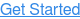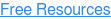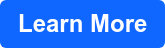# Master intuition and visualisation for VCE Physics

VCE Physics questions are usually packed with units, numbers and diagrams. You and I both know it’s easy to get lost in the information, misunderstand it or make silly errors when calculating the answer. That’s why it’s so important to hone your intuition – that is, a calculated ‘feeling’ for what the answer should or shouldn’t be.

Being able to visualise problems is another important skill. When you’re not given a diagram or graph, drawing out what’s going on can help you make key connections between all the information given, which can in turn lead you straight to the correct answer.

You might hear people say they type of intuition and visualisation useful for Physics is an ill-defined quality you either have or don’t have. From experience, I beg to differ! Here are some the ways I learned to develop my intuition and visualisation skills over time, ways that you can also incorporate in your own Physics practice.

### Apply units intuitively

‘But I don’t know the units for this!’ is a common refrain among Physics students doing practice questions, especially when starting a new topic. However, there are some neat tricks you can apply to help combat your confusion.

#### Equations, equations, equations

To build unit intuition and ensure we do always use the correct unit, a key clue is always hidden in the equation for the quantity.

For instance, we can instantly see the unit for momentum, p, through its equation:Momentum is the product of mass and velocity; accordingly, its unit will also be the product between the units of mass and velocity.

Being the difference between initial and final momentum, or p2 - p1, the change in momentum - impulse - will have units of kg ms-1 well.

However, there’s another unit for impulse floating around that tends to confuse students. But there’s a basis behind it hidden in the alternative equation for impulse, or Δp:This is why both Ns and kg ms-1 are acceptable VCAA units for momentum and impulse.

Applying these links between the equations and units for each quantity, we now only really have three SI units to memorise:

• Wb for magnetic flux
• J for mechanical energy (kinetic, gravitational, elastic)
• W for power

Not too bad now, is it?

#### Avoid imaginary units

Another issue I often see is students applying units when they shouldn’t be there. Lorentz factor, diffraction ratio, transformation equations (ratios)... In addition to their unitless states, these all have one thing in common: their equations all consist solely of fractions (or ratios) of comparable quantities. For example, the units for both and w in the diffraction ratio can be given in m. Consequently, the units of each quantity will cancel out, and we’ll be left with a unitless factor.

### Check using alternative methods

You were sure you had that ten-part Power Transmission question in your last SAC in the bag. Now the marks are out… and you see your work flooded with blood red crosses, 9/10 parts wrong. It’s easy to feel defeated when this happens, and the feedback you get from teachers and peers (“check your answers”, “be wary of the time”) can feel more hampering than helpful in situations like these.

However, a solid way to steer clear of this situation is to not just check your solutions and working out, but to check them using an alternative method.

Let’s apply this to a common VCAA-style question: “How much power is (or has been) lost in the transmission lines?” For this example, let’s say you’ve got a set of transmission lines with current 15 A, and total resistance 2 Ω.

How would we approach a question like this? If you thought Ploss = Iline2Rline, you would be correct!

We can quickly write this down and chuck it into our calculator like so.But there’s another way to do this that’s often overlooked, which you can use to check your initial answer. That’s to first calculate the voltage drop across the transmission line:And then use P = IV to calculate the power loss:Your teachers might have told you to be wary of using P = IV to calculate the power loss across transmission lines. That’s because you must use Vdrop to do this. However, used correctly, P = IV is a powerful tool: in one fell swoop, it can tell you whether your calculated values for total power, power loss, line current and line voltage (and thus resistance) are correct. This is super useful to kill two (or three or four) birds with one stone. Use it to see if your answers from multiple parts are correct: for instance, if you were asked to calculate the voltage drop across the transmission line in previous parts, on top of the power lost.

Over time, you might notice yourself starting to subconsciously recognise when values can click into place across the board like this. That’s your “intuition” helping you out. In the meantime, you’ve got a stellar method to instantly spot errors like these in a systematic manner.

### Visualise by grouping information

Diagrams are critical in VCE Physics, but it can be challenging to create a clear, helpful visual representation of the question at hand. Grouping information together can be huge quick fix, especially in fiddly Motion questions (since the acceleration of the system is equal to the acceleration of each separate component). This versatile technique can be applied to questions asking you about:

• Inclined planes
• Banked tracks
• Connected bodies
• Straight-line motion

Let’s see this applied to a question on connected bodies, courtesy of VCE Physics Exam 1, VCAA 2012.The first thing to always do with a question of this type is to visualise it by drawing in all the forces you can think of. To be systematic in my approach and help build my understanding of the problem, I draw in the forces in the same order each time:

1. Gravitational forces - always present on Earth!
2. Normal reaction forces - with surface contact: a must-have in inclined plane and banked track problems
3. Tension forces - present in string and connected bodies problems
4. Friction / resistive forces (if stated - most VCAA questions instruct us to ignore these)

In this case, there will be both gravitational and tension forces present. As the spheres are not in contact with any surfaces, there will be no normal reaction forces acting upon them.

We can draw these forces like so:We now approach the crux of the problem: grouping the spheres together.

While we could solve the problem by keeping the spheres separated and then calculating the magnitude of all of the forces using simultaneous equations like this:However, there’s a bit of a shortcut to be seen when we group the two spheres together as follows:As can be seen, the two gravitational forces of 2g and 1g add together, and the now ‘internal’ forces of TB cancel out. Now only two forces of TA and 3g act upon our mega-sphere. Applying Newton’s Second Law of Fnet = ma, we can identify:Keeping in mind that the spheres are merely “hanging” from the ceiling, we know that they are at rest. Hence, we know that they have a net acceleration of 0, and thus, a net force of 0 N acting upon them.Have a go at incorporating these techniques in your next Physics question. With a bit of work, little visualisation strategies such as these start to grow into your intuition. Eventually, you'll find yourself identifying what some forces and values are with nothing more than a glance.

### In summary

As I’ve said, I truly believe in nurture over nature when it comes to developing intuition and visualisation for Physics. By applying these tips to your own VCE Physics practice consistently, you will soon be able to see improvement as you solve complex problems with a more logical process, and pick up silly errors you otherwise would have missed. Even better, you’ll find that these great skills are fully transferable to other VCE Maths and Science subjects, and are therefore doubly worth acquiring, honing and mastering.## Connect lectures. #1 in VCE.

Unique and unlike any. Access gold-standard revision created and delivered by brilliant VCE graduates like Angela.## You May Also Like

These Stories on Exams